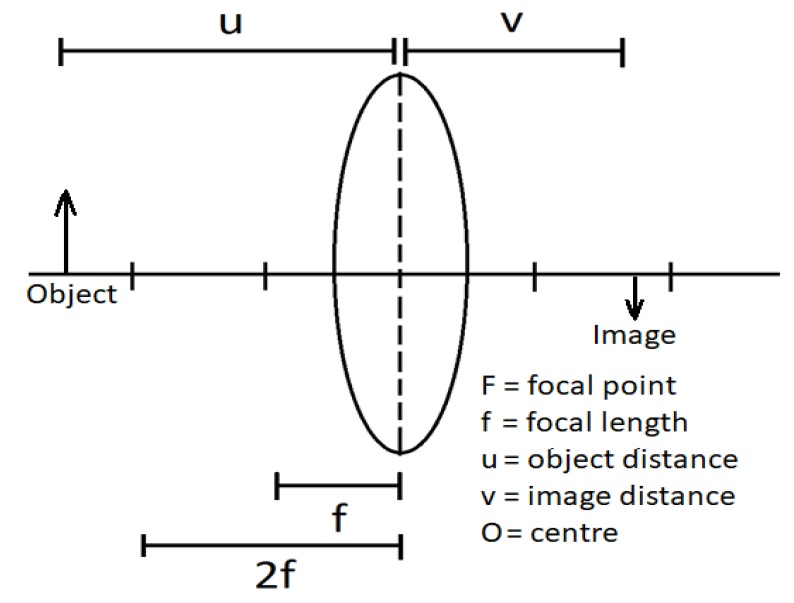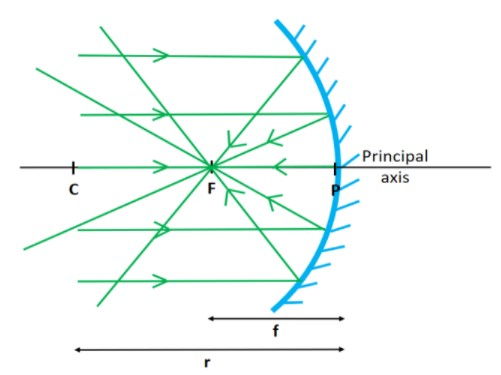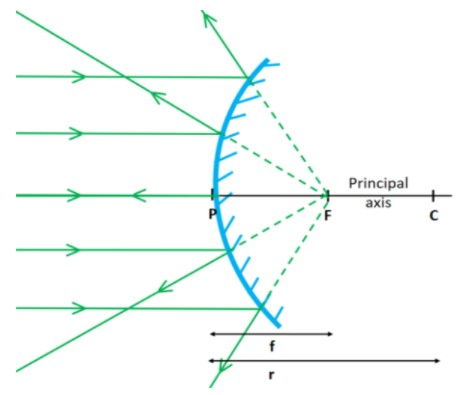## Light and Optics

This chapter is about refraction of light, total internal reflection, image formation by lenses, thin lens formula, optical instruments and image formation by spherical mirrors.

 6.1 Refraction of light

 Refraction of light The bending of a light ray at the boundary of two medium as the light ray propagates from a medium to another with difference optical density. The speed and direction of light changes when in different medium

• Light that moves from less dense to denser medium will refracted towards normal.
• Otherwise, the light that moves from denser to less dense medium will refracted away from normal.

 Formula for refraction index, n $$n=\dfrac{\text{sin i}^0}{\text{sin r}^0}$$,   (Snell's law), where $$\text{i}^0$$ = incidence angle, $$\text{r}^0$$ = refracted angle $$n=\dfrac{D}{d}$$, where D = real depth, d = apparent depth $$n=\dfrac{c}{v}$$,   where c = velocity of light in air ($$3\times10^8\text{ms}^{-1}$$), v = velocity of light in a medium $$n=\dfrac{1}{\text{sin c}^0}$$,   where $$\text{c}^0$$ = critical angle

 6.2 Total Internal Reflection

 Total Internal Reflection Reflection of light rays at the border of two medium when the angle of incidence in an optically denser medium is greater than the critical angle.

 The critical angle, c The maximum angle of incidence before the total internal reflection can occur ($$r^0=90^0$$)

Conditions for total internal reflection:

• i > c
• light moves from denser $$\rightarrow$$ less dense medium

Examples of total internal reflection:

• Mirage
• Prism periscope
• Prism binocular
• Fiber optics

 6.3 Image Formation by LensesCharacteristics of image

• Enlarged
• Diminished
• Same size
• Inverted
• Upright
• Virtual
• Real

Lenses

1. There are 2 types of lenses, namely the

• Convex lens

• Concave lens

2. Light rays passing through a convex or converging lens are bent towards the principal axis, whereas rays passing through a concave or diverging lens are bent away from the principal axis.

 Linear magnification, m Ratio of image height to object height (no unit) $$m=\dfrac{h_i}{h_o}$$, where $$h_i =$$ image height,  $$h_o =$$ object height $$m=\dfrac{v}{u}$$, where $$v =$$ image distance, $$u=$$ object distance

 6.4 Thin Lens Formula

 Lens equation relating $$f\,,u\,,v$$ $$\dfrac{1}{f}=\dfrac{1}{u}+\dfrac{1}{v}$$, where f = focal length, u = object distance, v = image distance

 $$+$$ $$-$$ $$f$$ convex lens (converging) concave lens (diverging) $$v$$ real image virtual image (same side with object)

 6.5 Optical Instruments

 Microscope Telescope Similarities Two convex lenses First image (the object of the eyepiece: reals, inverted Final image: virtual, inverted, magnified Differences Lens type: 2 high-powered lens Lens type: 1 high(eyepiece), 1 low-powered lens Focal length: $$f_0 < f_e$$ Focal length: $$f_0 > f_e$$ First image: enlarged First image: diminished Position of final image: close to the eye Position of final image: infinity Distance between the lens: $$D>f_0+f_e$$ Distance between the lens: $$D=f_0+f_e$$ Magnification: $$M=m_0\times m_e$$ Magnification: $$M=\dfrac{f_0}{f_e}$$

Applications of Small Lenses in Optical Instrument Technology

• small lenses are used in smartphone cameras and CCTV
• uses small size convex lens
• produce real, inverted and diminished image at the sensor
• to produce image with such characteristics (R, I, D) the distance between sensor and centre of lens has to be at least the length of focal length of lens

 6.6 Image Formation by Spherical Mirrors

Field of vision

• Plane mirror - narrow
• Concave mirror - very narrow
• Convex mirror - wide

 Concave mirror Convex mirror• C - Centre of curvature
• r - Radius of curvature
• F - Focal point
• f - Focal length
• u - Object distance
• v - Image distance

Applications of Concave Mirrors in Daily Life:

• Cosmetic mirror
• Dental mirror
• Reflector in car headlight

Applications of Convex Mirrors in Daily Life:

• Blind spot mirror
• Security mirror in buildings
• Vehicle rear mirror